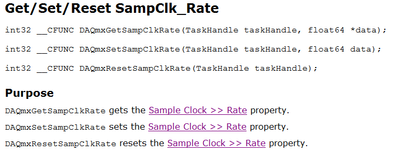# Multifunction DAQ

cancel
Showing results for
Did you mean:

## Determine the decimation rate (oversampling rate) programmatically

Hello all,

I am using the NI-DAQmx C API with a cDAQ-9185 controller and various modules with delta-sigma converters.

I can illustrate my problem with the NI-9232 IEPE module: According to the datasheet, the module supports three different "decimation rates" (= oversample rates): 64x, 128x and 256x.

My question: Is it possible to programmatically determine the current decimation rate (oversampling rate)?

As far as I can see, only the value DAQmx_SampClk_TimebaseDiv can be queried, which is the product of 2 times decimation rate times clock divider.

Thanks a lot!

Message 1 of 5
(602 Views)

## Re: Determine the decimation rate (oversampling rate) programmatically

Valid Sampling Rates for NI DSA, CM C Series and SC Express Devices

Use DAQmxGetSampClkRate to get the actual sampling rate.Message 2 of 5
(572 Views)

## Re: Re: Determine the decimation rate (oversampling rate) programmatically

Thank you, ZYOng, for your answer. Actually I'm not really referring to the sampling rate, but to the oversampling rate of the delta-sigma converter.

The datasheet of the 9232 module describes:Preliminary solution I implemented yesterday was:

``````double oversamplingRate = 0.;

// first get base and sampling rate
float64 timeBaseRate = 0.;
float64 samplingRate = 0.;

DAQmxGetSampClkTimebaseRate(taskHandle, &timeBaseRate)); // get time base
DAQmxGetSampClkRate(taskHandle, &samplingRate));         // get sampling rate as set by driver

// compute decimation factor
const int decimationFactor = static_cast<int>(timeBaseRate / samplingRate);

// decimationFactor = 2 x decimatinRate x clockDivider, clockDivider is in [1, 26] (cf. 9232 datasheet)
const int cMinClockDivider = 1;
const int cMaxClockDivider = 26;

// possible decimation rates for Ni-9232 oversampling
const std::set<int> sDecimationRates = { 64, 128, 256 }; // cf. 9232 specs

// now find a decimation rate that fulfills samplingRate = baseRate / (2 x decimationRate x clockDivider)
bool decimationRateFound = false;

for (auto it = sDecimationRates.crbegin(), itEnd = sDecimationRates.crend(); it != itEnd; ++it) {
const int decimationRate = *it;
const int potentialClockDivider = decimationFactor / 2 / decimationRate;
if ((potentialClockDivider >= cMinClockDivider) && (potentialClockDivider <= cMaxClockDivider)) {
decimationRateFound = true;
oversamplingRate = decimationRate*samplingRate; // now we got it!
break;
}
}
``````

However, this solution has two disadvantages:

1. It is valid only for the 9232 module
2. I do not think that the solution is unique.

As for the second point, I choose the highest possible oversampling rate, but I don't know what NI does internally.

So I've been looking for an API function to query the oversampling rate, but I don't think there is one.

Message 3 of 5
(556 Views)

## Re: Re: Determine the decimation rate (oversampling rate) programmatically

And why is the decimation rate important to the user if is handled by the driver?

Santhosh
Soliton Technologies

Finding it hard to source NI hardware? Try NI Trading Post
Message 4 of 5
(544 Views)

## Re: Re: Re: Determine the decimation rate (oversampling rate) programmatically

@santo_13 wrote:

And why is the decimation rate important to the user if is handled by the driver?

I need the decimation rate to determine the input delay:

Cf. https://www.ni.com/docs/de-DE/bundle/ni-9232-specs/page/overview.html:Message 5 of 5
(540 Views)